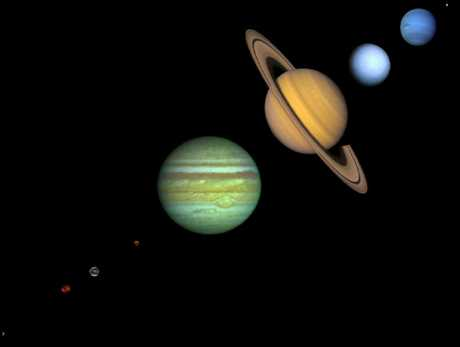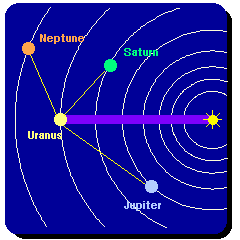Gravitational Perturbations
and the Prediction of New Planets

Computing the orbit of the Earth as an ellipse around the center of mass for the Earth-Sun system assumes that they are the only two masses in the Universe. In reality, the Universal Law of Gravitation implies that the Earth interacts gravitationally not only with the Sun, but with every other mass in the Universe: the Moon, the other planets, asteroids and comets, the distant stars.

## The Two-Body Approximation

However, from the form of the gravitational forcewe see that the interactions are largest when two situations are fulfilled: (1) the product of the masses of the two objects is large, which maximizes the numerator of the expression for the strength of the gravitational force, and (2) the objects are near each other, which minimizes the denominator of the force equation. The two-body approximation that the orbits of the planets are determined only by the gravitational interaction between the Sun and the planet is possible because
1. The Sun is so massive compared with every other object in the Solar System,

2. Objects outside the Solar System such as stars are so distant that the distance squared factor in the denominator renders their gravitational interactions with the planets negligible.
For example, the Sun is about 300,000 times more massive than the Earth, and about 1000 times more massive than the largest planet. Thus, the product of the mass of a planet and the mass of the Sun is always much larger than the product of the masses of any two planets, and it is a good initial approximation to neglect all interactions except that of the planet and the Sun.

## Gravitational Perturbations

However, the small deviations from this ideal picture have consequences if careful measurements are made. These small deviations from the simplified picture are called perturbations. They can be calculated systematically using Newton's laws of motion and gravitation from the positions of the known masses in the Solar System.

If we account carefully for all known gravitational perturbations on the motion of observed planets and the motion of the planet still deviates from the prediction, there are two options:

1. Newton's Law of Gravitation requires modification,

2. There is a previously undetected mass that is perturbing the orbits of the observed planets.
We shall see that the history of astronomy following the introduction of the Law of Gravitation by Newton gives examples of both.

## The Discovery of the New Planet NeptuneIn 1846, the planet Neptune was discovered after its existence was predicted because of discrepancies between calculations and data for the planet Uranus. Astronomers found the new planet almost exactly at the position predicted by the calculations of Leverrier (Adams had also calculated the position independently). We illustrate the situation schematically in the adjacent diagram. The dominant interaction between Uranus and the Sun is indicated with the heavy line, but some perturbations associated with other masses are indicated by thin lines. By using Newton's laws to calculate the perturbations on the orbit of Uranus by an hypothesized new planet, Leverrier and Adams were able to predict where the planet had to be in order to cause the observed deviations in the position of Uranus. Once astronomers took this calculation seriously, they found the new planet within hours of turning their telescopes on the region of the sky implicated by the calculations.

This precise prediction of the new planet and its location was striking confirmation of the power of Newton's theory of gravitation. (Although in truth it must be said that both Leverrier and Adams made an incorrect assumption in their calculations concerning the radius of the new planet's orbit. Fortunately, the error largely cancelled out of the calculations and had little effect on their final results.)

## The Accidental Discovery of Pluto

Later, similar calculations on supposed perturbations of the orbits of Uranus and Neptune suggested the presence of yet another planet beyond the orbit of Neptune. Eventually, in 1930, a new planet Pluto was discovered, but we now know that the calculations in this case were also in error because of an incorrect assumption about the mass of the new planet. It is now believed that the supposed deviations in the orbits of Neptune and Uranus were errors in measurement because the actual properties of Pluto would not have accounted for the supposed perturbations. Thus, the discovery of Pluto was a kind of accident.

## Effects Beyond Newtonian Perturbations

The power of Newton's theory became apparent as detailed calculations accounted more and more precisely for the orbits of the planets. Any deviations from the expected behavior soon became viewed as evidence for unseen masses in the Solar System. However, later observations of anomalies in the orbit of Mercury could not be accounted for by the gravitational perturbation of a new planet (the hypothetical new planet, which turned out not to exist, was called Vulcan). As we discuss in the next section, early in this century this forced the replacement of Newton's Law of Gravitation with Einstein's Theory of General Relativity.